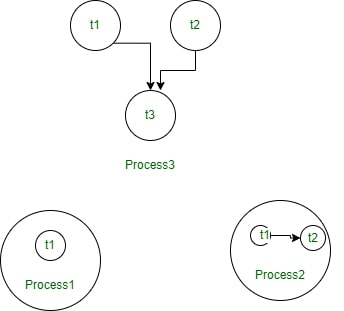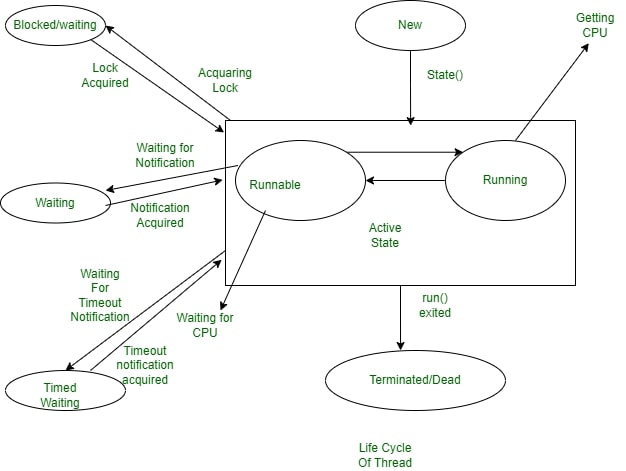GeeksforGeeks App
Open AppBrowser
Continue

## Related Articles

Typically, we can define threads as a subprocess with lightweight with the smallest unit of processes and also has separate paths of execution. These threads use shared memory but they act independently hence if there is an exception in threads that do not affect the working of other threads despite them sharing the same memory.Threads in a Shared Memory Environment in OS

As we can observe in, the above diagram a thread runs inside the process and there will be context-based switching between threads there can be multiple processes running in OS, and each process again can have multiple threads running simultaneously. The Multithreading concept is popularly applied in games, animation…etc.

To help users Operating System accommodates users the privilege of multitasking, where users can perform multiple actions simultaneously on the machine. This Multitasking can be enabled in two ways:

In this type of Multitasking, processes are heavyweight and each process was allocated by a separate memory area. And as the process is heavyweight the cost of communication between processes is high and it takes a long time for switching between processes as it involves actions such as loading, saving in registers, updating maps, lists, etc.

As we discussed above Threads are provided with lightweight nature and share the same address space, and the cost of communication between threads is also low.

Now, we can understand why threads are being used as they had the advantage of being lightweight and can provide communication between multiple threads at a Low Cost contributing to effective multi-tasking within a shared memory environment.

There are different states Thread transfers into during its lifetime, let us know about those states in the following lines: in its lifetime, a thread undergoes the following states, namely:

1. New State
2. Active State
3. Waiting/Blocked State
4. Timed Waiting State
5. Terminated StateWe can see the working of  different states in a Thread in the above Diagram, let us know in detail each and every state:

1. New State

By default, a Thread will be in a new state,  in this state, code has not yet been run and the execution process is not yet initiated.

2. Active State

A Thread that is a new state by default gets transferred to Active state when it invokes the start() method, his Active state contains two sub-states namely:

• Runnable State: In This State, The Thread is ready to run at any given time and it’s the job of the Thread Scheduler to provide the thread time for the runnable state preserved threads. A program that has obtained Multithreading shares slices of time intervals which are shared between threads hence, these threads run for some short span of time and wait in the runnable state to get their schedules slice of a time interval.
• Running State: When The Thread Receives CPU allocated by Thread Scheduler, it transfers from the “Runnable” state to the “Running” state. and after the expiry of its given time slice session, it again moves back to the “Runnable” state and waits for its next time slice.

3. Waiting/Blocked State

4. Timed Waiting State

Sometimes the longer duration of waiting for threads causes starvation, if we take an example like there are two threads T1, T2 waiting for CPU and T1 is undergoing a Critical Coding operation and if it does not exist the CPU until its operation gets executed then T2 will be exposed to longer waiting with undetermined certainty, In order to avoid this starvation situation, we had Timed Waiting for the state to avoid that kind of scenario as in Timed Waiting, each thread has a time period for which sleep() method is invoked and after the time expires the Threads starts executing its task.

5. Terminated State

A thread will be in Terminated State, due to the below reasons:

• Termination is achieved by a Thread when it finishes its task Normally.
• Sometimes Threads may be terminated due to unusual events like segmentation faults, exceptions…etc. and such kind of Termination can be called Abnormal Termination.
• A terminated Thread means it is dead and no longer available.

As we are familiar, we create Main Method in each and every Java Program, which acts as an entry point for the code to get executed by JVM, Similarly in this Multithreading Concept, Each Program has one Main Thread which was provided by default by JVM, hence whenever a program is being created in java, JVM provides the Main Thread for its Execution.

### How to Create Threads using Java Programming Language?

We can create Threads in java using two ways, namely :

2. Implementing a Runnable interface

We can run Threads in Java by using Thread Class, which provides constructors and methods for creating and performing operations on a Thread, which extends a Thread class that can implement Runnable Interface. We use the following constructors for creating the Thread:

## Java

 `import` `java.io.*;``import` `java.util.*;` `public` `class` `GFG ``extends` `Thread {``    ``// initiated run method for Thread``    ``public` `void` `run()``    ``{``        ``System.out.println(``"Thread Started Running..."``);``    ``}``    ``public` `static` `void` `main(String[] args)``    ``{``        ``GFG g1 = ``new` `GFG();` `        ``// Invoking Thread using start() method``        ``g1.start();``    ``}``}`

Output

`Thread Started Running...`

Sample code to create Thread by using Runnable Interface:

## Java

 `import` `java.io.*;``import` `java.util.*;` `public` `class` `GFG ``implements` `Runnable {``    ``// method to start Thread``    ``public` `void` `run()``    ``{``        ``System.out.println(``            ``"Thread is Running Successfully"``);``    ``}` `    ``public` `static` `void` `main(String[] args)``    ``{``        ``GFG g1 = ``new` `GFG();``        ``// initializing Thread Object``        ``Thread t1 = ``new` `Thread(g1);``        ``t1.start();``    ``}``}`

Output

`Thread is Running Successfully`

## Java

 `import` `java.io.*;``import` `java.util.*;` `public` `class` `GFG {``    ``public` `static` `void` `main(String args[])``    ``{``        ``// Thread object created``        ``// and initiated with data``        ``Thread t = ``new` `Thread(``"Hello Geeks!"``);` `        ``// Thread gets started``        ``t.start();` `        ``// getting data of``        ``// Thread through String``        ``String s = t.getName();``        ``System.out.println(s);``    ``}``}`

Output

`Hello Geeks!`

Sample Java Code which creates Thread Object by using Thread(Runnable r, String name):

## Java

 `import` `java.io.*;``import` `java.util.*;` `public` `class` `GFG ``implements` `Runnable {``    ``public` `void` `run()``    ``{``        ``System.out.println(``            ``"Thread is created and running successfully..."``);``    ``}``    ``public` `static` `void` `main(String[] args)``    ``{``        ``// aligning GFG Class with``        ``// Runnable interface``        ``Runnable r1 = ``new` `GFG();``        ``Thread t1 = ``new` `Thread(r1, ``"My Thread"``);``        ``// Thread object started``        ``t1.start();``        ``// getting the Thread``        ``// with String Method``        ``String str = t1.getName();``        ``System.out.println(str);``    ``}``}`

Output

```My Thread
Thread is created and running successfully...
```

Java Program to explore different Thread States:

Let us see the working of thread states by implementing them on Threads t1 and t2.

Output:

## Java

 `import` `java.io.*;``import` `java.util.*;` `class` `GFG ``implements` `Runnable {``    ``public` `void` `run()``    ``{``        ``// implementing try-catch Block to set sleep state``        ``// for inactive thread``        ``try` `{``            ``Thread.sleep(``102``);``        ``}``        ``catch` `(InterruptedException i1) {``            ``i1.printStackTrace();``        ``}``        ``System.out.println(``            ``"The state for t1 after it invoked join method() on thread t2"``            ``+ ``" "` `+ ThreadState.t1.getState());` `        ``// implementing try-catch block``        ``try` `{``            ``Thread.sleep(``202``);``        ``}``        ``catch` `(InterruptedException i2) {``            ``i2.printStackTrace();``        ``}``    ``}``}` `// creation of ThreadState class``// to implement Runnable interface``public` `class` `ThreadState ``implements` `Runnable {``    ``public` `static` `Thread t1;``    ``public` `static` `ThreadState o1;``    ``public` `static` `void` `main(String args[])``    ``{``        ``o1 = ``new` `ThreadState();``        ``t1 = ``new` `Thread(o1);``        ``System.out.println(``"post-spanning, state of t1 is"``                           ``+ ``" "` `+ t1.getState());``        ``// lets invoke start() method on t1``        ``t1.start();``        ``// Now,Thread t1 is moved to runnable state``        ``System.out.println(``            ``"post invoking of start() method, state of t1 is"``            ``+ ``" "` `+ t1.getState());``    ``}``    ``public` `void` `run()``    ``{``        ``GFG g1 = ``new` `GFG();``        ``Thread t2 = ``new` `Thread(g1);``        ``// Thread is created and its in new state.``        ``t2.start();``        ``// Now t2 is moved to runnable state``        ``System.out.println(``            ``"state of t2 Thread, post-calling of start() method is"``            ``+ ``" "` `+ t2.getState());``        ``// create a try-catch block to set t1 in waiting``        ``// state``        ``try` `{``            ``Thread.sleep(``202``);``        ``}``        ``catch` `(InterruptedException i2) {``            ``i2.printStackTrace();``        ``}``        ``System.out.println(``            ``"State of Thread t2 after invoking to method sleep() is"``            ``+ ``" "` `+ t2.getState());``        ``try` `{``            ``t2.join();``            ``System.out.println(``                ``"State of Thread t2 after join() is"``                ``+ ``" "` `+ t2.getState());``        ``}``        ``catch` `(InterruptedException i3) {``            ``i3.printStackTrace();``        ``}``        ``System.out.println(``            ``"state of Thread t1 after completing the execution is"``            ``+ ``" "` `+ t1.getState());``    ``}``}`

Output

```post-spanning, state of t1 is NEW
post invoking of start() method, state of t1 is RUNNABLE
state of t2 Thread, post-calling of start() method is RUNNABLE
The state for t1 after it invoked join method() on thread t2 TIMED_WAITING
State of Thread t2 after invoking to method sleep() is TIMED_WAITING
State of Thread t2 after join() is TERMINATED
state of Thread t1 after completing the execution is RUNNABLE```

My Personal Notes arrow_drop_up